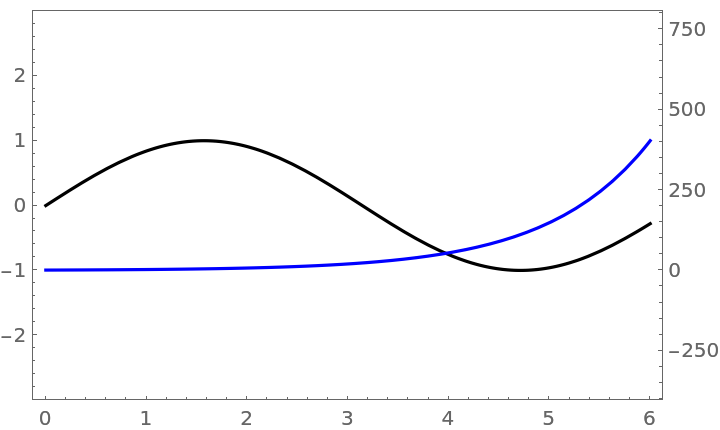#Function Repository Resource:

# MultipleAxesPlot

Display different vertical axes for two plotted expressions

Contributed by: Jon McLoone  |  Jon McLoone
 ResourceFunction["MultipleAxesPlot"][{expr1,expr2},{x,xmin,xmax}] generates a plot of expr1 and expr2 with two y-axes.

## Details and Options

ResourceFunction["MultipleAxesPlot"] supports all of the options of Plot except Axes, ScalingFunctions, Frame, Ticks, TicksStyle and FrameTicks.

## Examples

### Basic Examples (1)

Plot two functions with different scales:

 In:=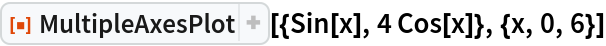Out=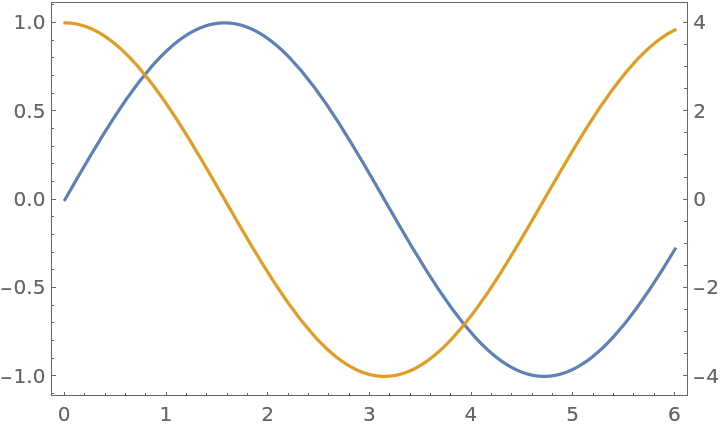### Options (3)

To make it clearer which axes refers to which line, you can use "SecondaryAxesColor":

 In:=Out=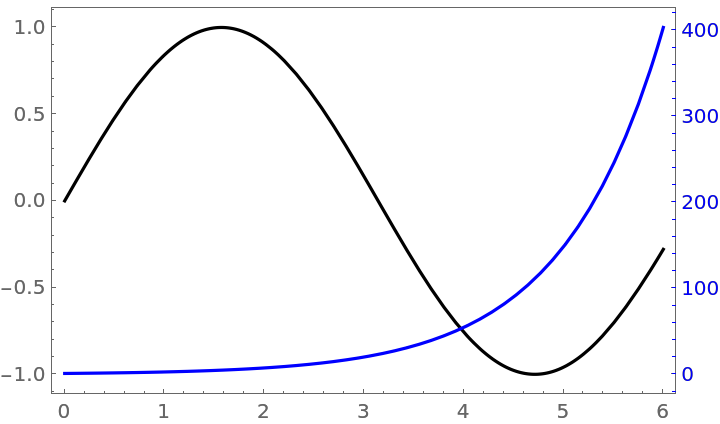You can control the plot ranges of the two lines using PlotRange and "SecondaryPlotRange":

 In:=Out=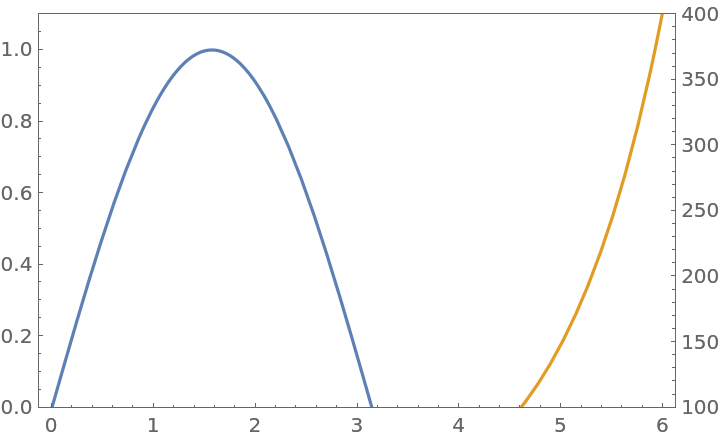PlotRangePadding applies to both functions but uses the coordinate system of the first function:

 In:=Out=